JEE  >  Physics Test 8 - Electrostatics, Capacitance, Current Electricity, Magnetic Effect, Magnetism, Emi And AC

# Physics Test 8 - Electrostatics, Capacitance, Current Electricity, Magnetic Effect, Magnetism, Emi And AC

Test Description

## 25 Questions MCQ Test JEE Main & Advanced Mock Test Series | Physics Test 8 - Electrostatics, Capacitance, Current Electricity, Magnetic Effect, Magnetism, Emi And AC

Physics Test 8 - Electrostatics, Capacitance, Current Electricity, Magnetic Effect, Magnetism, Emi And AC for JEE 2023 is part of JEE Main & Advanced Mock Test Series preparation. The Physics Test 8 - Electrostatics, Capacitance, Current Electricity, Magnetic Effect, Magnetism, Emi And AC questions and answers have been prepared according to the JEE exam syllabus.The Physics Test 8 - Electrostatics, Capacitance, Current Electricity, Magnetic Effect, Magnetism, Emi And AC MCQs are made for JEE 2023 Exam. Find important definitions, questions, notes, meanings, examples, exercises, MCQs and online tests for Physics Test 8 - Electrostatics, Capacitance, Current Electricity, Magnetic Effect, Magnetism, Emi And AC below.
Solutions of Physics Test 8 - Electrostatics, Capacitance, Current Electricity, Magnetic Effect, Magnetism, Emi And AC questions in English are available as part of our JEE Main & Advanced Mock Test Series for JEE & Physics Test 8 - Electrostatics, Capacitance, Current Electricity, Magnetic Effect, Magnetism, Emi And AC solutions in Hindi for JEE Main & Advanced Mock Test Series course. Download more important topics, notes, lectures and mock test series for JEE Exam by signing up for free. Attempt Physics Test 8 - Electrostatics, Capacitance, Current Electricity, Magnetic Effect, Magnetism, Emi And AC | 25 questions in 60 minutes | Mock test for JEE preparation | Free important questions MCQ to study JEE Main & Advanced Mock Test Series for JEE Exam | Download free PDF with solutions
 1 Crore+ students have signed up on EduRev. Have you?
Physics Test 8 - Electrostatics, Capacitance, Current Electricity, Magnetic Effect, Magnetism, Emi And AC - Question 1

### Two charges of magnitude +Q and two charges of magnitude -q are placed at the opposite corners of a square of side length ‘a’. If the resultant force on a charge Q is zero than

Physics Test 8 - Electrostatics, Capacitance, Current Electricity, Magnetic Effect, Magnetism, Emi And AC - Question 2

### Work done in carrying a charge ‘Q’ from ‘A’ to ‘B’ (as shown in figure) on a circle of radius ‘r’ with a charge ‘Q’ at the centre is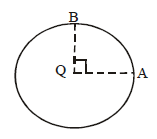Detailed Solution for Physics Test 8 - Electrostatics, Capacitance, Current Electricity, Magnetic Effect, Magnetism, Emi And AC - Question 2 A circle around a point charge represents an equipotential surface (V= Kq/r throughout the perimeter), and work done on an equipotential surface is zero.
Physics Test 8 - Electrostatics, Capacitance, Current Electricity, Magnetic Effect, Magnetism, Emi And AC - Question 3

### Two spheres having radii of 5 cm and 10 cm bear identical charge of 6.6 nC. Find the potential of spheres after they have been connected by a conductor, assume that the spheres are at large distance from each other

Physics Test 8 - Electrostatics, Capacitance, Current Electricity, Magnetic Effect, Magnetism, Emi And AC - Question 4

An ideal electrical cell does 5 joule of work in carrying 10 coulomb of charge around a closed circuit. The heat loss or energy consumption in the circuit outside the battery is

Physics Test 8 - Electrostatics, Capacitance, Current Electricity, Magnetic Effect, Magnetism, Emi And AC - Question 5

A charged particle of mass 0.1 g is in equilibrium in an electric field E = 98 N/C. The charge on the particle is

Physics Test 8 - Electrostatics, Capacitance, Current Electricity, Magnetic Effect, Magnetism, Emi And AC - Question 6

An electric charge ‘q’ is lying at the open end of a cylindrical vessel. The flux coming out of the cylinder will be

Detailed Solution for Physics Test 8 - Electrostatics, Capacitance, Current Electricity, Magnetic Effect, Magnetism, Emi And AC - Question 6 If you put a another cylinder on it then charge will completely in side and flux will distribute half half so flux =q/2εₒ
Physics Test 8 - Electrostatics, Capacitance, Current Electricity, Magnetic Effect, Magnetism, Emi And AC - Question 7

On moving a charge of 20 coulombs by 2cm, 2J of work is done, then the potential difference between the points is

Detailed Solution for Physics Test 8 - Electrostatics, Capacitance, Current Electricity, Magnetic Effect, Magnetism, Emi And AC - Question 7 Va - Vb =W/q.
W= work done :2Jq= charge : 20C Va - Vb = 2/20 = 0.1
Physics Test 8 - Electrostatics, Capacitance, Current Electricity, Magnetic Effect, Magnetism, Emi And AC - Question 8

The capacitance of a parallel plate condenser does not depend upon

Physics Test 8 - Electrostatics, Capacitance, Current Electricity, Magnetic Effect, Magnetism, Emi And AC - Question 9

A semi-circular are of radius ‘a’ is charged uniformly and the charge per unit length is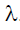. The electric field at its centre is

Physics Test 8 - Electrostatics, Capacitance, Current Electricity, Magnetic Effect, Magnetism, Emi And AC - Question 10

Which of the following cannot be the units of electric intensity?

Physics Test 8 - Electrostatics, Capacitance, Current Electricity, Magnetic Effect, Magnetism, Emi And AC - Question 11

The unit of permittivity of free space ∈0  is

Detailed Solution for Physics Test 8 - Electrostatics, Capacitance, Current Electricity, Magnetic Effect, Magnetism, Emi And AC - Question 11

Solution :- By coulomb's law the electrostatic force

F = 1/4πε0(q1q2/r2)

⇒ε0 = 1/4πF(q1q2/r2)

Substituting the unit for q, r,and F , we abtain unit of

ε0 = coulomb×coulomb newton−(metre)2

= (coulumb)2 newton −(meter)2

=C2N−m2

Physics Test 8 - Electrostatics, Capacitance, Current Electricity, Magnetic Effect, Magnetism, Emi And AC - Question 12

Ampere hour is a unit of

Physics Test 8 - Electrostatics, Capacitance, Current Electricity, Magnetic Effect, Magnetism, Emi And AC - Question 13

Six resistors each of value 10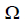, are connected to a 5 V battery as shown in the figure. The reading of ammeter'A' is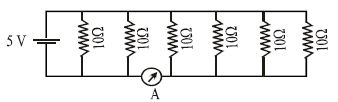Physics Test 8 - Electrostatics, Capacitance, Current Electricity, Magnetic Effect, Magnetism, Emi And AC - Question 14

The potential difference between points ‘A’ and ‘B(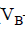-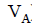)' in the figure if R=0.7is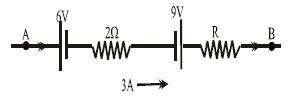Detailed Solution for Physics Test 8 - Electrostatics, Capacitance, Current Electricity, Magnetic Effect, Magnetism, Emi And AC - Question 14 Ans) Applying Kirchhoff's first law
Va - 6 - 2(3) + 9 - (0.7)(3) =Vb
Vb - Va = 9- 6 - 6 - 2.1
=- 3 - 2.1
= - 5.1
Physics Test 8 - Electrostatics, Capacitance, Current Electricity, Magnetic Effect, Magnetism, Emi And AC - Question 15

If 2% of the main current is to be passed through the galvanometer of resistance G. the resistance of shunt required is

Detailed Solution for Physics Test 8 - Electrostatics, Capacitance, Current Electricity, Magnetic Effect, Magnetism, Emi And AC - Question 15
Let total current through the circuit be I.
Then, 0.02I current is to be passed through galvanometer of resistance G.
Let the resistance of shunt is Rs .
∵ Shunt are in parallel combination with Galvanometer.
∴ 0.02I * G = (1 - 0.02)I * Rs
⇒0.02G = 0.98 * Rs
⇒Rs = 0.02G/0.98 = G/49

Physics Test 8 - Electrostatics, Capacitance, Current Electricity, Magnetic Effect, Magnetism, Emi And AC - Question 16

The thermo e.m.f. of a thermocouple is given by e = 2164 t - 6.2 t2 . The neutral temperature and a temperature of inversion are

Physics Test 8 - Electrostatics, Capacitance, Current Electricity, Magnetic Effect, Magnetism, Emi And AC - Question 17

The relation between Faraday constant F, electron charge e, and Avogadro's Number N is

Physics Test 8 - Electrostatics, Capacitance, Current Electricity, Magnetic Effect, Magnetism, Emi And AC - Question 18

In the circuit as shown in Fig. The heat produced by 6resistance due to current flowing in it is 60 calories per second. The heat generated across 3 ohm resistance per second will be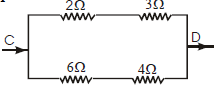Physics Test 8 - Electrostatics, Capacitance, Current Electricity, Magnetic Effect, Magnetism, Emi And AC - Question 19

A thin bar magnet of length 2l and breadth 2b, pole strength p and magnetic moment M is divided into four equal parts with length and breadth of each part being half of the original magnet. Then the pole strength of each part is

Physics Test 8 - Electrostatics, Capacitance, Current Electricity, Magnetic Effect, Magnetism, Emi And AC - Question 20

Susceptibility is positive and small for a ........substance:

*Answer can only contain numeric values
Physics Test 8 - Electrostatics, Capacitance, Current Electricity, Magnetic Effect, Magnetism, Emi And AC - Question 21

A battery of internal resistance 4 ohm is connected to the network of resistance as shown. In the order that the maximum power can be delivered to the network, the value of R in ohm should be :-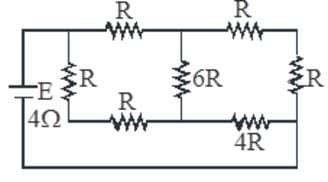Detailed Solution for Physics Test 8 - Electrostatics, Capacitance, Current Electricity, Magnetic Effect, Magnetism, Emi And AC - Question 21

Condition for maximum power is
r = R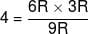R = 2

*Answer can only contain numeric values
Physics Test 8 - Electrostatics, Capacitance, Current Electricity, Magnetic Effect, Magnetism, Emi And AC - Question 22

A capacitor of capacitance 5 μF is connected as shown in the fig. The internal resistance of the cell is 0.5 Ω. The amount of charge on the capacitor plate is :- (μC) :-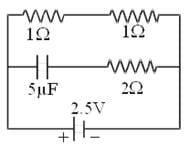*Answer can only contain numeric values
Physics Test 8 - Electrostatics, Capacitance, Current Electricity, Magnetic Effect, Magnetism, Emi And AC - Question 23

Consider two concentric non-conducting uniformly charged spherical shells of radius 3m and 6m having charges 10 µC and 200 µC respectively. Find the interaction potential energy between two spheres in joules.

Detailed Solution for Physics Test 8 - Electrostatics, Capacitance, Current Electricity, Magnetic Effect, Magnetism, Emi And AC - Question 23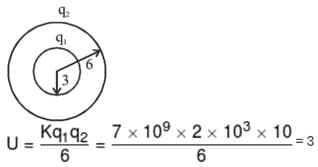*Answer can only contain numeric values
Physics Test 8 - Electrostatics, Capacitance, Current Electricity, Magnetic Effect, Magnetism, Emi And AC - Question 24

Diagram shows a part of circuit. Find VA–VB when current I is 5A and it is decreasing at a rate of 10+3 A/s ?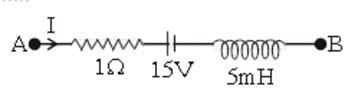Detailed Solution for Physics Test 8 - Electrostatics, Capacitance, Current Electricity, Magnetic Effect, Magnetism, Emi And AC - Question 24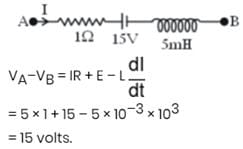*Answer can only contain numeric values
Physics Test 8 - Electrostatics, Capacitance, Current Electricity, Magnetic Effect, Magnetism, Emi And AC - Question 25

A current of 4 A flows in a coil when connected to a 12 V d.c. source. If the same coil is connected to a 12 V, 50 rad/s, a.c. source, a current of 2.4 A flows in the circuit. Determine the reactance (in Ω) of coil.

Detailed Solution for Physics Test 8 - Electrostatics, Capacitance, Current Electricity, Magnetic Effect, Magnetism, Emi And AC - Question 25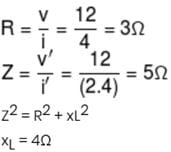## JEE Main & Advanced Mock Test Series

2 videos|325 docs|189 tests
 Use Code STAYHOME200 and get INR 200 additional OFF Use Coupon Code
Information about Physics Test 8 - Electrostatics, Capacitance, Current Electricity, Magnetic Effect, Magnetism, Emi And AC Page
In this test you can find the Exam questions for Physics Test 8 - Electrostatics, Capacitance, Current Electricity, Magnetic Effect, Magnetism, Emi And AC solved & explained in the simplest way possible. Besides giving Questions and answers for Physics Test 8 - Electrostatics, Capacitance, Current Electricity, Magnetic Effect, Magnetism, Emi And AC, EduRev gives you an ample number of Online tests for practice

## JEE Main & Advanced Mock Test Series

2 videos|325 docs|189 tests